# nodal curve

## higher

last updated: 2003-10-18When a is a rational number, the curve is an algebraic curve.
Each curve consists of equal branches, that merge in a rotation of p/a.

La Gournerie studied the curve in 1851.

Some specific specimen are the following: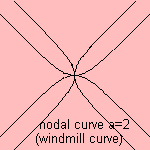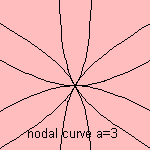For a=1, the kappa curve, a quartic, results.
For a=2 we see the windmill curve, a sextic 1).
For a being integer, the power of the curve is 2*a +2.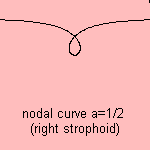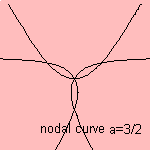For a=1/2, you find the straight strophoid.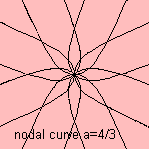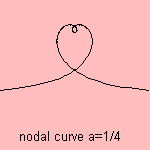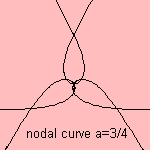notes

1) With formula: x2y2 (x2 + y2) = (x2 - y2)2